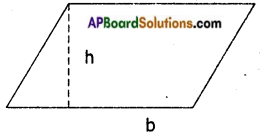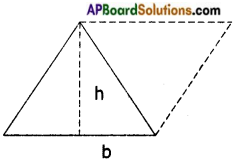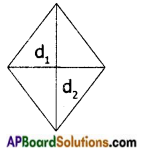Students can go through AP Board 7th Class Maths Notes Chapter 13 Area and Perimeter to understand and remember the concepts easily.

## AP State Board Syllabus 7th Class Maths Notes Chapter 13 Area and Perimeter

→ The area of a parallelogram is equal to the product of its base (b) and corresponding height (h) A = bh.Any side of the parallelogram can be taken as its base.

→ The area of a triangle is equal to half the product of its base and height.A = $$\frac{1}{2}$$ bh
A triangle = Half a parallelogram

→ The area of a Rhombus is equal to half the product of Its diagonals.A = $$\frac{1}{2}$$ d1d2

→ The circumference of a circle = 2πr = πd where π = $$\frac{22}{7}$$ or 3.14, d = $$\frac{r}{2}$$